Courses

# Differential Amplifiers

## 10 Questions MCQ Test Topicwise Question Bank for Electronics Engineering | Differential Amplifiers

Description
This mock test of Differential Amplifiers for Electronics and Communication Engineering (ECE) helps you for every Electronics and Communication Engineering (ECE) entrance exam. This contains 10 Multiple Choice Questions for Electronics and Communication Engineering (ECE) Differential Amplifiers (mcq) to study with solutions a complete question bank. The solved questions answers in this Differential Amplifiers quiz give you a good mix of easy questions and tough questions. Electronics and Communication Engineering (ECE) students definitely take this Differential Amplifiers exercise for a better result in the exam. You can find other Differential Amplifiers extra questions, long questions & short questions for Electronics and Communication Engineering (ECE) on EduRev as well by searching above.
QUESTION: 1

### A resitive loaded and biased differential amplifier circuit is shown in figure. Neglecting the base currents and assuming matched transistors with VA = ∞,β = 100, w hat are the values of Rc and RE to meet the following specifications? Differential mode gain (double ended) = -500, Common mode rejection ratio = 500, Differential mode input resistance = 2 MQ.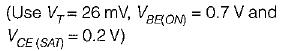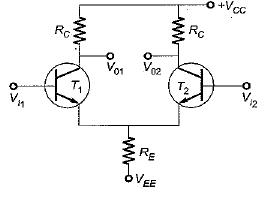Solution: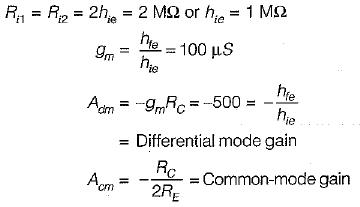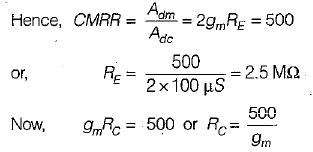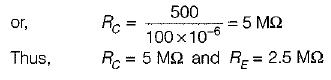QUESTION: 2

### In the level shifter circuit shown in figure beiow, the internal drop across each diode VD = VBE2 = 0.7 V and hfe is very large. The value of R for V0 to become zero is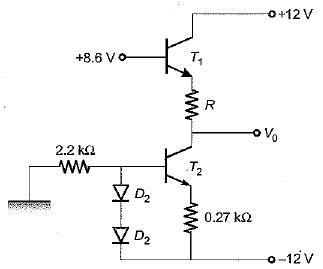Solution:

The aiven circuit is redrawn as shown below.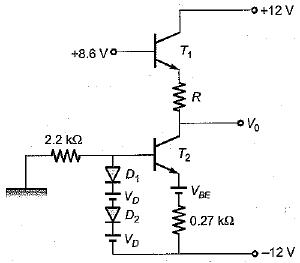Writing loop equation containing two diodes and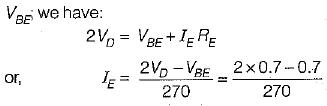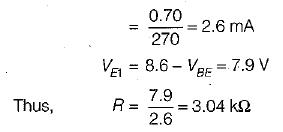QUESTION: 3

### A differential amplifier, amplifies

Solution:

The goal of designing differential amplifiers is to minimize the effect of common-mode input signal and amplify the difference of the voltages between the two input lines.

QUESTION: 4

Most of linear ICs are based on the two transistor differential amplifier because of its

Solution:
QUESTION: 5

A differential amplifier has RL = 10 kΩ(equal values in both collectors), hie = 1 kΩ, Re = 50 kΩ, hte = 100. The common mode gain is given by

Solution:

Common- mode gain,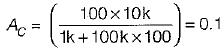QUESTION: 6

In a single-stage differential amplifier, the output offset voltage is basically dependent on the mismatch of

Solution:

In single stage differential amplifier, the output offset voltage is basically dependent on the mismatch of IB and β.

QUESTION: 7

Assertion (A): It is not so easy to design d.c. amplifiers using transistors.
Reason (R): The values of hfe ,VBE and ICBO vary with temperature.

Solution:

The values of hFE, VBE and ICB0 vary with temperature and due to the variation in any of these quantities the output voltage may change which may not be distinguished from a change in the input signal voltage, Due to this reason it is not so easy to design d.c. amplifiers using transistors. Hence, both assertion and reason are true and reason is the correct explanation of assertion.

QUESTION: 8

Assertion (A): The ability of a differential amplifier to reject a differential mode signal is called its “figure of merit”.
Reason (R): The ideal value of figure of merit of a differential amplifier is infinite.

Solution:

Assertion is not true because “figure of merit” (or CM MR) of a differential amplifier is to reject a common mode signal (vC).

QUESTION: 9

A differential amplifier has (i) CMMR= 1000 and (ii) CMRR= 10000.
The first set of inputs is V1= 100 μV and V2, = -100μV.
The second set of inputs is V1= 1100 μV and V2 = 900 μV.
What is the percentage difference in output voltage obtained for the two sets of input voltages?

Solution:

we know that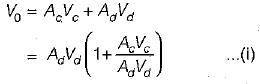Now,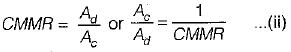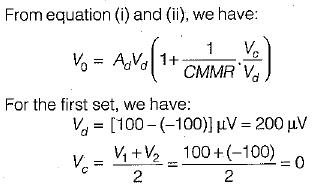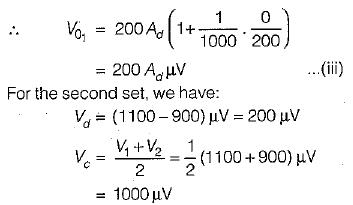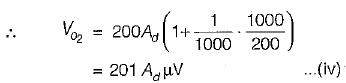From equation (iii) and (iv), the percentage difference in output voltage obtained for the two sets of input voltages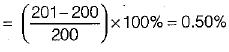QUESTION: 10

The Q-point (Vc and IB) for the differential amplifier shown in figure below is given by: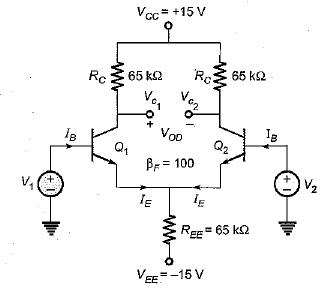Solution: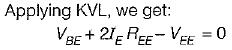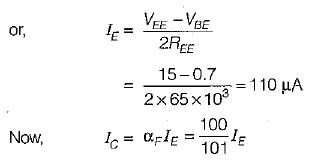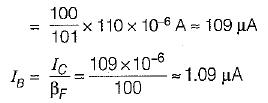Also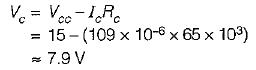Hence, both transistors of the differential amplifier are biased at a Q-point having lB = 1.09 μA and Vc=7.9V.